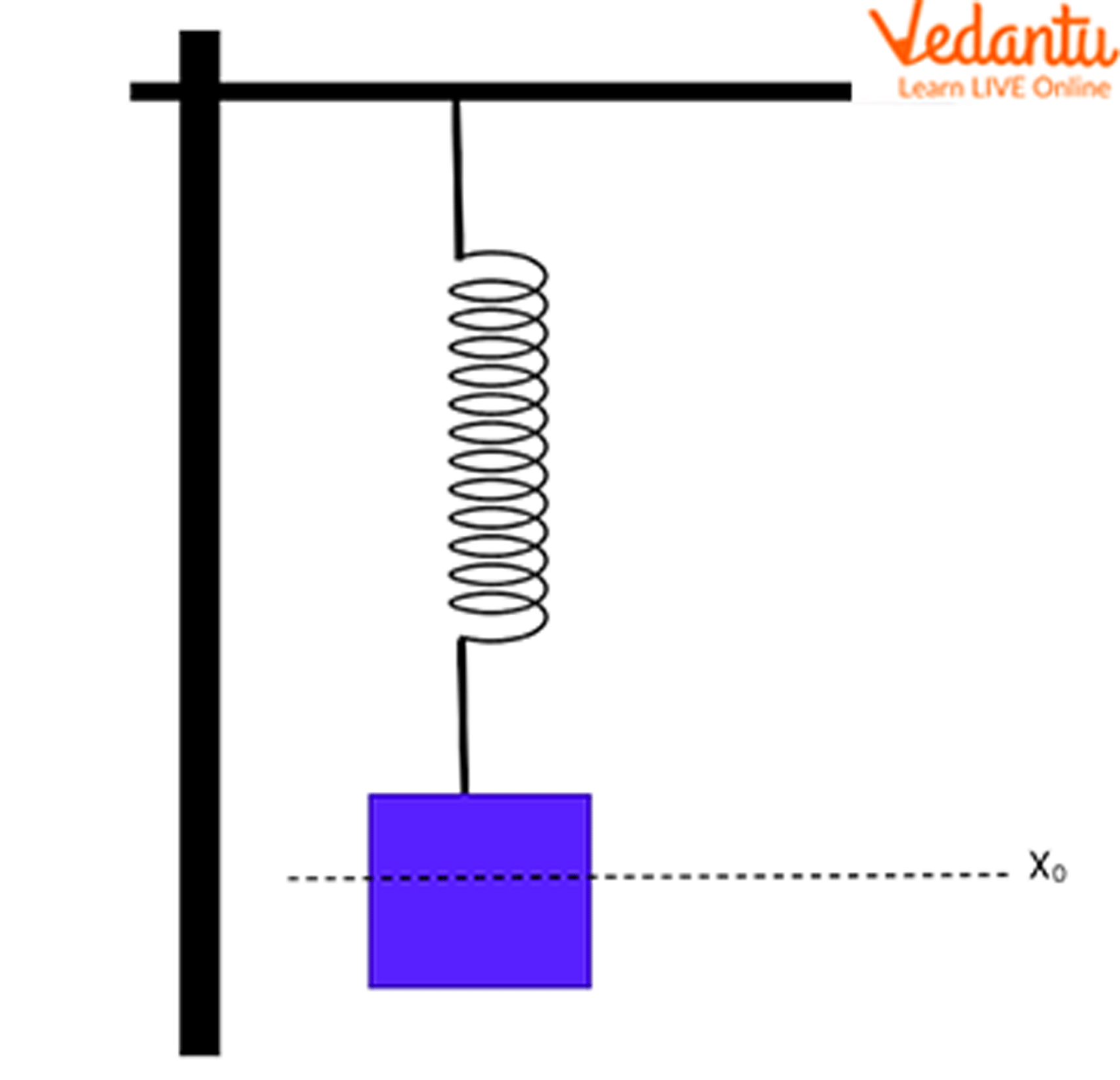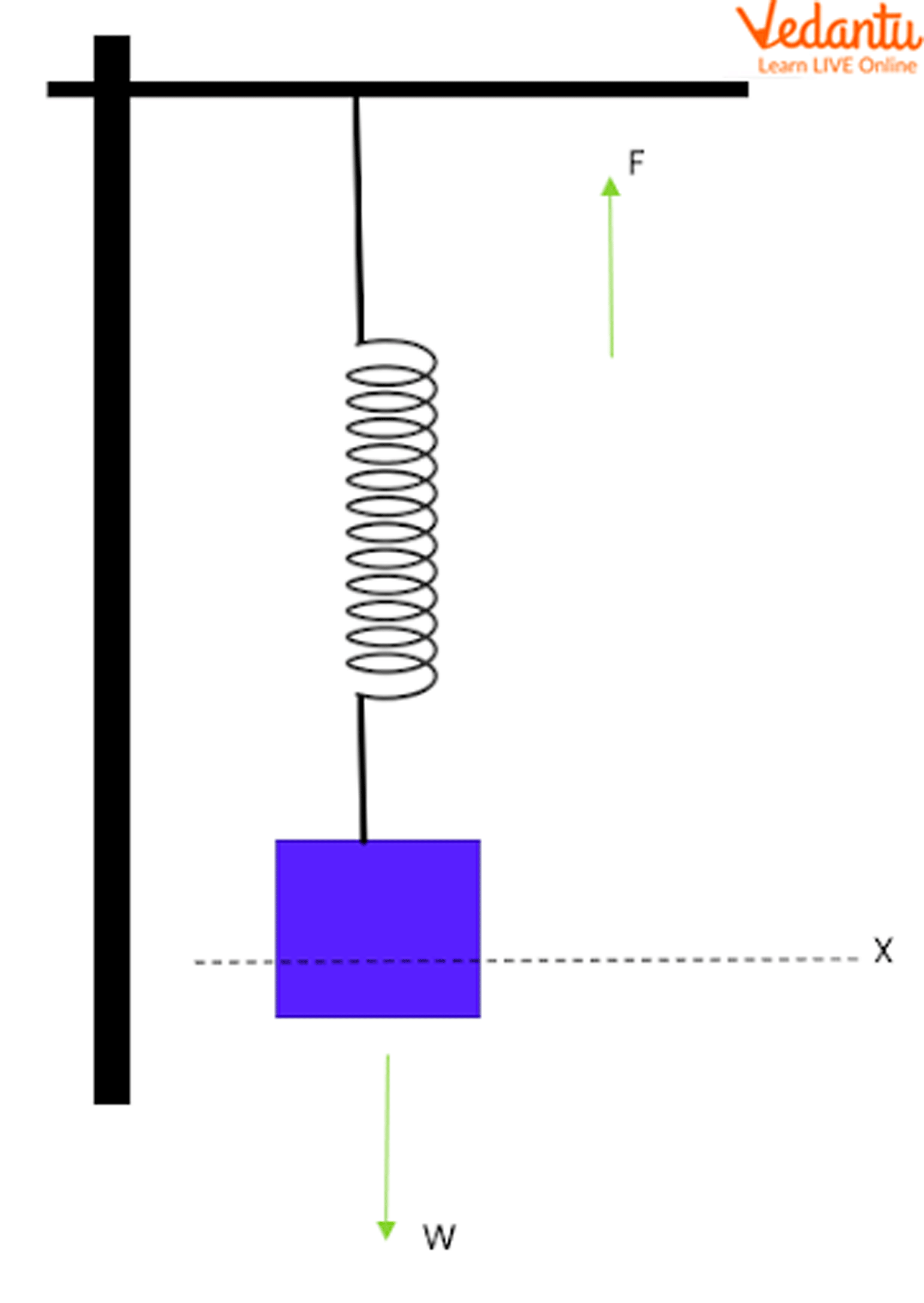Courses
Courses for Kids
Free study material
Free LIVE classes
More

# Spring Force - Important Concepts with Examples for JEELIVE
Join Vedantu’s FREE Mastercalss

## A Glance on Springs and The Spring Force

Generally, we use springs widely in our day-to-day life. These are the elastic substances which can store the mechanical energy and are made of spring steel. These are referred to as coil springs by many people who are using springs.

When a load is attached to the hook of a spring, a load force comes into play which results in the compression or a stretch from their original resting positions.

When the spring is stretched or compressed, a so-called spring force will be acting on the spring which opposes the load force. These springs are categorised into many types based on how this load force is applied to them. For Example, Tension springs and Compression springs. As their names say, tension springs are the springs which get stretched when a load force is applied to them. And compression springs work only when a compression force is applied and the spring gets compressed to its length. Let’s discuss more how spring force works, its work done and the spring force examples.

## What is Spring Force?

Let us assume a load of mass ‘m’ is attached to a tension spring and is resting at an equilibrium position named xo. Let us demonstrate this with a diagram.Load Resting on a Spring at an Equilibrium Position

Now due to the weight of the load acting on the spring, it gets stretched downwards and causes a displacement of x in the downward direction only. Let us demonstrate the position of the spring with another diagram.Spring Force Opposing the Weight

As we can see from the diagram, F is acting in the opposite direction of W.

Let us write the change in positions or displacement of the load from Xo to X as

(X - X0)

The spring force acts in such a way that it opposes the change in displacement of the load which is resting in an equilibrium position. Therefore, it is directly proportional to the change in displacement of the load.

I.e., $F\propto (X-X_0)$

Therefore, the formula of force in a spring is given by the spring force equation,

• F= -k(X - X0)

Where K is known as the spring constant or a force constant which varies from spring to spring based on the material the spring is made with.

• X0 is the position where the load is in equilibrium with the spring

• X is the position displacement from the equilibrium position

Here as we can see, the spring force acts opposingly to the weight of the load. This is why it has a negative sign in the spring force equation. This force equation is also known as Hooke’s Law in Physics. Here, the spring force acts opposite to the change in the position of the load attached to it. It means that the spring is trying to restore the position of the load to equilibrium despite the other forces acting on it. This is why this is termed the restoring force.

Let us look into the spring force examples that are used widely in our lives.

• The springs have been used majorly in manufacturing toys since the late 18th century.

• Spring balance is used to measure the weights using a spring.

• Also, in medical equipment, electrical appliances and in pendulums we can see the huge usage of springs. In shock absorbers, brakes and clutches, springs are used as short coil springs to hold the friction and driven plates together and absorb the tensional vibrations in them. Now let us see the work done by the spring force formula.

## Work Done by Spring Force

To find the work done by the spring force, we assume two different positions of the load, which are

Let the initial position of the load be X1 and the final position be X

The work done by a force is given by the equation,

W = F.x

As we know, the force can be changing like a variable, let’s take the integral of the spring force to obtain the equation for work done.

Let the variable force be F(x) and therefore apply the integral,

$W_{s}=\int F(x) d x$

We put the limits of the integral from the initial position of the load X1 to the final position of the load X

$W_{s}=\int_{X_{1}}^{X_{2}} F(x) d x$

Substituting F(x) = -kx in the above equation, we get

$W_{s}=\int_{X_{1}}^{X_{2}}-k x \cdot dx=-k\left[\dfrac{x^{2}}{2}\right]_{X_{1}}^{X_{2}}$

Therefore, solving the above equation gives us the final result as,

• $W_{s}=\dfrac{1}{2} k\left(x_{1}^{2}-\mathrm{x}_{2}^{2}\right)$

This is the work done by spring force formula and if we assume the initial position as the equilibrium point, then x1 becomes zero.

Therefore,

• $W_{s}=-\dfrac{1}{2} k x^{2}$

This is the equation for work done by spring force and here

• Ws  is the spring work

• x  is the displacement of the spring from the equilibrium to a specific position and k is the spring constant.

Let us solve an example here.

Example: Find the work done by the spring of the displacement due to the spring force being 5m and the spring constant is 4.2$\dfrac{N}{m}$.

Ans: As we know the equation for work done by the spring is

$W=-\dfrac{1}{2} k x^{2}$

Given that, x = 5m and k = 4.2$\dfrac{N}{m}$

Therefore, Work done = –(0.5)(4.2)(5)2 = – 52.5 Nm

Here, the minus sign indicates that it is opposing the change in spring’s displacement.

Therefore, we derived the equations for the spring load calculation and the work done by the spring force calculation.

## Conclusion

Thereby, we learned restoring force definition, and how to define the term force in springs with a derivation and a valid explanation. The formula of force in springs is used in physics where we use a spring balance or a pendulum. Spring is a substance that can store elastic potential energy within itself by pressuring the atomic bonds in an elastic material. This spring has a wide variety of applications in a human’s life.

Last updated date: 17th Sep 2023
Total views: 135.3k
Views today: 1.35k

## FAQs on Spring Force - Important Concepts with Examples for JEE

1. What are the various types of springs and how are they classified?

There are various kinds of springs classified based on the load force applied to them such as tension spring, compression spring and the torsion spring. Tension springs work only when a stretching force is applied to the load. Compression springs operate when the compression force is applied and the spring gets shorter. Torsion springs are those which operate only if a twisting force is applied to the spring. Also, there are springs which are categorised based on the material they are made up of. For example, coil springs, moulded springs and many more.

2. What is the weightage of this topic in JEE?

The spring constant and the elasticity concept have a weightage of about 4% in JEE. This is also related to the work and energy concept which is very important in JEE. A maximum of 1 question can be asked on this topic and it can be related to finding the displacement in the spring, finding the spring constant, finding the spring force, and finding the work done by the spring force. Learning this concept is very useful in solving all the above problems.

3. What is Hooke’s Law?

The equation of spring force F = -kx is also known as Hooke’s Law. Basically, this law states that Force with which a spring pushes back or pulls forward is directly proportional to the displacement of the load from its initial position. There is a limit for every elastic material beyond which if it is extended or compressed, it will not come back to its original state. Some materials will not obey Hooke’s Law if they are stretched or compressed beyond their elastic limit. This law can be used to define the term force in springs and also for restoring force definition.